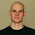## 29 August 2009

### 4 equals 5In a previous post, I showed that 4 = 3. Now this proof from Futility Closet demonstrates that 4 also equals 5.

If this interests you, now might be a good time to dig into TYWKIWDBI's (reasonably brief) math section.

1.The error is in step 2. Factoring the fractions should result in 4*(1/4) on the left and 5*(1/5) on the right.

2.3.if this equation was correct, then every number we know would equal other numbers-any number, that is!

4.oops error.............

5.Nice try, but that doesn't work i'm afraid - and the other examples on Futility Closet don't work either, they're all forgetting something or misusing a maths step.

Or this is all just a joke?

6.7.The maths is faulty.

8.it's not even that fun. it's like saying pig is meat, cow is meat, therefore pig is cow. It's just stupid. There are better and more interesting examples of proving falseties.

9.Ev and others - this puzzle (and the other ones at the links) aren't meant to be taken seriously. It's a form of (geeky) humor.

10.Well.... ok! :)

I'm glad though. And the fun bit was me trying to figure out what the wrong bit was.

Also, to me it makes point in social commentary: how people can be fooled and not accepting mathematical truths.

To get serious, I would bring up examples of Creationists falsely misinterpreting science to make argue against scientific theories/facts (delibrately or not). This is what I thought when I saw these puzzles.

But maybe I'm taking this too far - it was just a joke ;)

11.but actually 4 does equal 5 haha:
-20=-20
-36+16=-45+25
-36+16-(81/4)=-45+25-(81/4)
(4-(9/2))^2=(5-(9/2))^2
4-(9/2)=5-(9/2)
4=5